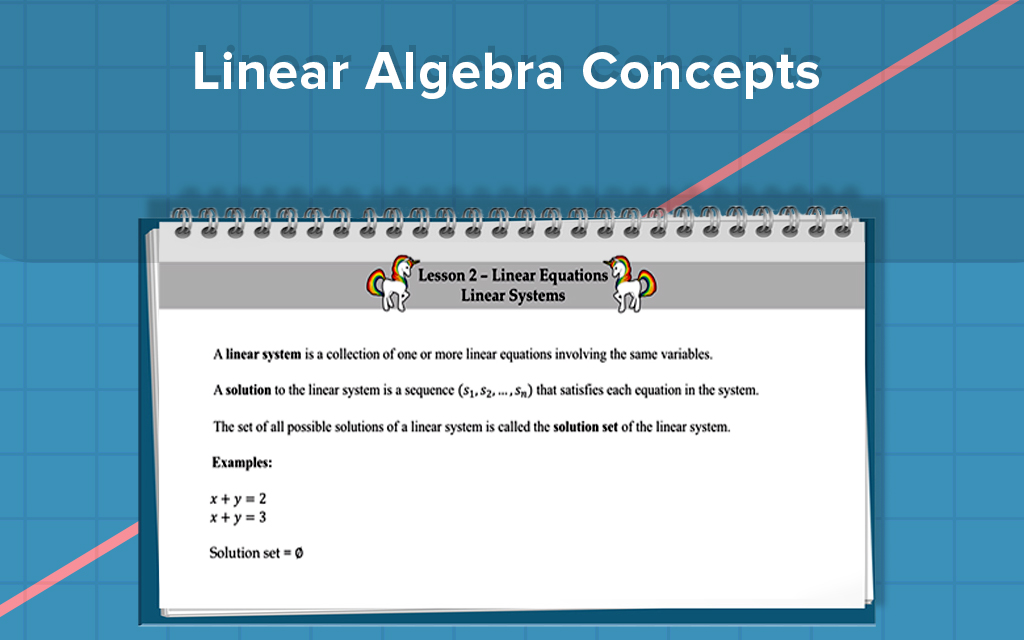## FREE

This course includes:

•1 hr 22 mins of video courses
•Full lifetime access
•Go at your own pace
•Certificate of completion

Stay up to date:

What you'll learn

Students will learn how to solve linear systems of equations and answer theoretical questions about linear systems

Requirements

Knowledge of basic algebra concepts is helpful.Best seller

# Overcoming Linear Algebra Concepts

Jun 07 2021 - Video Course (1 hr 22 mins)

This course will help you develop a deeper understanding of the theory of linear equations, linear systems, equivalent systems, echelon form, reduced row echelon forms, row reduction and gain the mathematical knowledge and skill level required to answer questions about linear systems. This course will teach you how to solve linear systems using elimination methods, Gaussian elimination, and Gauss Jordan reduction.

Created by Steve Warner

Linear Algebra

## FREE

This course includes:

•1 hr 22 mins of video courses
•Full lifetime access
•Go at your own pace
•Certificate of completion

Stay up to date:

What you'll learn

Students will learn how to solve linear systems of equations and answer theoretical questions about linear systems

Requirements

Knowledge of basic algebra concepts is helpful.

## Course Content

1 Unit - 8 video lessons
What is a Linear Equation?
In this video, you will learn about linear equations in two variables, three variables, and four variables with examples. Sometimes, we use the word ‘unknowns’ instead of the word ‘variable’. I will also give you examples of nonlinear equations, and you will learn about a solution to linear equations with examples.
06:01
Linear Systems
A linear system is a collection of one or more linear equations involving the same variables. A solution to the linear system is a sequence that satisfies each equation in the system. In this video, you will learn about the linear systems with examples, solutions to the linear system with examples, and the solution set of a linear system.
08:39
The Elimination Method
The elimination method is useful for solving small linear systems. In particular, the elimination method works really well for solving systems of two equations with two variables. In this video, you will learn how to solve linear equations using elimination methods with examples.
09:17
10:53
Echelon Form
A matrix is in row echelon form if it satisfies three conditions. In this video, you will learn about the three conditions satisfied for a matrix to be in row echelon form with examples.
11:46
Row Reduction
Row reduction is a 4-step algorithm used to produce a matrix in row echelon form. This algorithm is also known as Gaussian elimination. A 5th step can be added to produce a matrix in reduced row echelon form. In this case, the algorithm is called Gauss-Jordan Reduction. In this video, I will use an example to walk you through the algorithm of row reduction. I will show you how to do both Gaussian elimination and Gauss-Jordan reduction.
12:04
More Examples of Row Reduction
In this video, I am going to go through 2 more examples of the row reduction algorithm by following the rules step-by-step.
10:58### Steve Warner

Pianist and Test Prep Expert

Dr. Steve Warner, a New York native, is a mathematician, test prep expert, pianist, and songwriter. Dr. Warner earned his Ph.D. at Rutgers University in Pure Mathematics in May 2001. While a graduate student, Dr. Warner won the TA Teaching Excellence Award. Dr. Warner is currently an Associate Professor of Mathematics at Hofstra University. He has taught undergraduate and graduate courses in Precalculus, Calculus, Linear Algebra, Differential Equations, Mathematical Logic, Set Theory, and Abstract Algebra. From 2003 – 2008, Dr. Warner participated in a five-year NSF grant, “The MSTP Project,” to study and improve mathematics and science curriculum in poorly performing junior high schools. He also published several articles in scholarly journals, specifically on Mathematical Logic. Dr. Warner has more than two decades of experience in general math tutoring and tutoring for standardized tests such as the SAT, ACT, GRE, GMAT, and AP Calculus exams. He has tutored students both individually and in group settings. Additionally, Dr. Warner has written many test prep books including his "28 SAT Math Lessons" and "28 ACT Math Lessons" series. He has also written several books in pure mathematics such as "Pure Mathematics for Beginners."

0 Reviews 0 Students 2 Courses